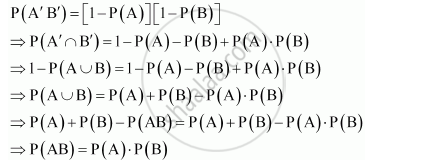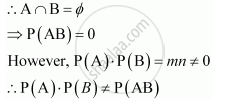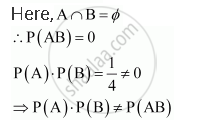# Two Events a and B Will Be Independent, If - Mathematics

Two events A and B will be independent, if

(A) A and B are mutually exclusive

(B) P(A'B') = [1 - P(A)][1-P(B)]

(C) P(A) = P(B)

(D) P(A) + P(B) = 1

#### Solution

Two events A and B are said to be independent, if P(AB) = P(A) × P(B)

Consider the result given in alternative B.This implies that A and B are independent, if  P(A'B') = [1 - P(A)][1-P(B)]

Distracter Rationale

A. Let P (A) = m, P (B) = n, 0 < mn < 1

A and B are mutually exclusiveC. Let A: Event of getting an odd number on throw of a die = {1, 3, 5}

P(A) = 3/6 = 1/2

B: Event of getting an even number on throw of a die = {2, 4, 6}

P(B) = 3/6 = 1/2D. From the above example, it can be seen that,

P(A) + P(B) = 1/2 + 1/2 = 1

However, it cannot be inferred that A and B are independent.

Thus, the correct answer is B.

Concept: Independent Events
Is there an error in this question or solution?

#### APPEARS IN

NCERT Class 12 Maths
Chapter 13 Probability
Q 18 | Page 548